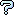All about flooble | fun stuff | Get a free chatterbox | Free JavaScript | Avatarsperplexus dot infoA Power of an Integer Beginning with an Arbitrary Sequence (Posted on 2008-01-31)L is any integer other than a power of 10. M is any integer. Show that there is an integral power of L that begins with the sequence of digits given in M.

 Submitted by FrankM Rating: 3.8000 (5 votes) Solution: (Hide) Map the real line onto a circle with circumference 1 (so that real numbers differing by an integer are sent to the same point). Next consider the sequence J Log10(L) for J=1,... Each element of this sequence is irrational, so that no two elements get mapped to the same point. If we consider the set of points with J up to J* = 1/Log10(1 + 1/M). Then there must be some pair J1>J2 both less than J* +1 such that J1 and J2 map to a pair of points separated by less than Log10(1 + 1/M). Next, consider the subsequence where J3 = J2 + (J2 - J1); J4 = J3 + (J2 - J1) etc. This subsequence marches around the circle in steps of less than Log10(1 + 1/M), so that for some JK it must come within Log10(1 + 1/M) of the point Log10(M). In other words: { Log10(M) } <= { JK * Log10(L) } < { Log10(M+1) }, where { } indicates "fractional part". This power, JK, satisfies our condition.Subject Author Datere: Might be the solution Charlie 2008-02-01 10:56:26Might be the solution Praneeth 2008-02-01 02:54:01Might be the solution Praneeth 2008-02-01 02:54:00Warning! A Hint from the Author FrankM 2008-01-31 20:56:35probabilistic solution Charlie 2008-01-31 14:04:02A possible exception... Dej Mar 2008-01-31 12:55:15Please log in:

 Search: Search body:
Forums (0)